Home Previous Next
5. A Switching Method
Consider the following 4x4 2D filters:

Filter1

Let xi,j i,j =1,2,3,4 be the pixel of the input to the filter.

y = output of the filter = x3,3

Filter2

Let z1 = average of x2,3 x3,2 and x3,3

z2 = average of x2,2 x3,2 and x3,3

z3 = average of x2,2 x2,3 and x3,3

z4 = average of x2,2 x2,3 and x3,2

y = median of z1 z2 z3 and z4

Filter3

This is a linear filter with weight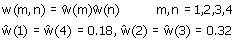Filter1 is the one in section3. And filter3 is similar to the Gaussian filter described in section2. Filter2 is somewhere in between filter1 and filter3. We want to apply filter1 to the region with edges to preserve detail and apply filter3 to the high frequency region to reduce aliasing and noise. Here is the idea of how we decide when to use which filter.
Let's look at the following 4x4 inputs.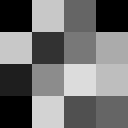input1 input2
We can guess that input1 may be an edge in the image while input2 has some high frequency. So we would like to use filter1 for input1 and filter3 for input2 but how can we distinguish these two patterns?

Among 16 input pixels, there are 24 pairs of pixels which share the same edge, as denoted by blue line in the figure below.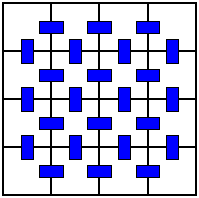In each pair, the difference between pixel values of input1 is smaller than that of input2 (on average). Denote the sum of this difference by D, we shall use the following parameter to decide which filter to use for a particular input.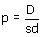where sd is the standard deviation of the pixel values. Our thresholds are:

If p < 18, use filter1.

If 18 < p < 22, use filter2.

If p > 22, use filter3.

For the interpolator, we use the weighted median one described in section3. The result is shown below.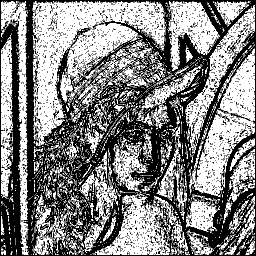First subsampled filtered image (g1)
Filter used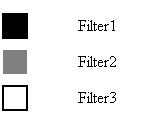Reconstructed Image
The switching method can capture edges in the image fairly well. The reconstructed image still have some blurred region due to the low-pass filter. The resulting PSNR and bit-rate are presented in the table below, compared to the former schemes.
 PSNR (dB) bit-rate (bit/pixel) Burt/Adelson 32.77 0.8278 Subsampling and weighted median interpolator 34.00 0.6544 Proposed method 33.58 0.6501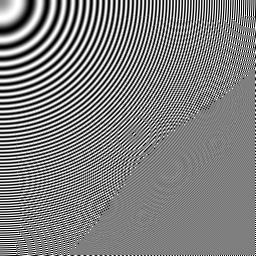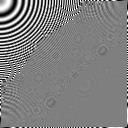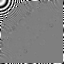g1 g2 g3
Gaussian pyramid of the proposed method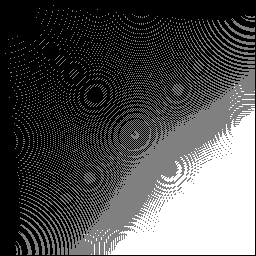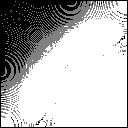Filter used
These are funny images, but it shows that the switching method works as we want. Linear low-pass filter is applied at the high frequency region while edge-preserving filter is used at the low frequency area.
 Home Previous Next